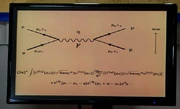FANDOM

1,364 PagesPictorial representations of particle interactions known as Feynman diagrams permeate many areas of theoretical physics. Theoretical physics is a branch of physics which employs both mathematical models and abstractions of physics in an attempt to explain natural phenomena. Its central core is mathematical physics, though other conceptual techniques are also used. The goal is to rationalize, explain and predict physical phenomena. The advancement of science depends in general on the interplay between experimental studies and theory. In some cases, theoretical physics adheres to standards of mathematical rigor while giving little weight to experiments and observations. For example, while developing special relativity, Albert Einstein was concerned with the Lorentz transformation which left Maxwell's equations invariant, but was apparently uninterested in the Michelson-Morley experiment on Earth's drift through a Luminiferous_aether| luminiferous ether. On the other hand, Einstein was awarded the Nobel Prize for explaining the photoelectric effect, previously an experimental result lacking a theoretical formulation.

For a list of theoretical physicists in The Big Bang Theory, view the Theoretical Physicists Category.

Overview

A physical theory is a model of physical events. It is judged by the extent to which its predictions agree with empirical observations. The quality of a physical theory is also judged on its ability to make new predictions which can be verified by new observations. A physical theory differs from a mathematical theorem in that while both are based on some form of axioms, judgment of mathematical applicability is not based on agreement with any experimental results.

$\mathrm{Ric} = k\,g$ The equations for an Einstein manifold, used in general relativity to describe the curvature of spacetime

A physical theory involves one or more relationships between various measurable quantities. Archimedes realized that a ship floats by displacing its mass of water, Pythagoras understood the relation between the length of a oscillation or vibrating string and the musical tone it produces, and how to calculate the dimension or length of a rectangle's diagonal. Other examples include entropy as a measure of the uncertainty regarding the positions and motions of unseen particles and the quantum mechanical idea that (action and) energy are not continuously variable.

Sometimes the vision provided by pure mathematical systems can provide clues to how a physical system might be modeled; e.g., the notion, due to Bernhard_Riemann| Riemann and others, that space itself might be curved.

Theoretical advances may consist in setting aside old, incorrect paradigms (e.g., Burning consists of evolving phlogiston, or Astronomical bodies revolve around the Earth) or may be an alternative model that provides answers that are more accurate or that can be more widely applied.

Physical theories become accepted if they are able to make correct predictions and no (or few) incorrect ones. The theory should have, at least as a secondary objective, a certain economy and elegance mathematical beauty), a notion sometimes called "Occam's razor" after the 13th-century English philosopher William of Occam (or Ockham), in which the simpler of two theories that describe the same matter just as adequately is preferred. (But conceptual simplicity may mean mathematical complexity.) They are also more likely to be accepted if they connect a wide range of phenomena. Testing the consequences of a theory is part of the scientific method.

Physical theories can be grouped into three categories: mainstream theories, proposed theories and fringe theories.